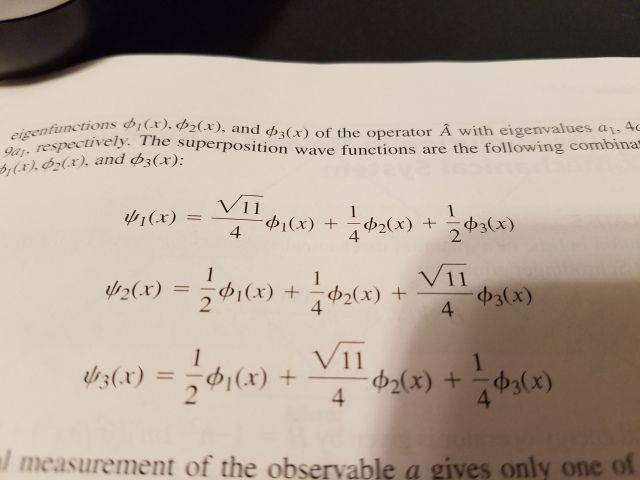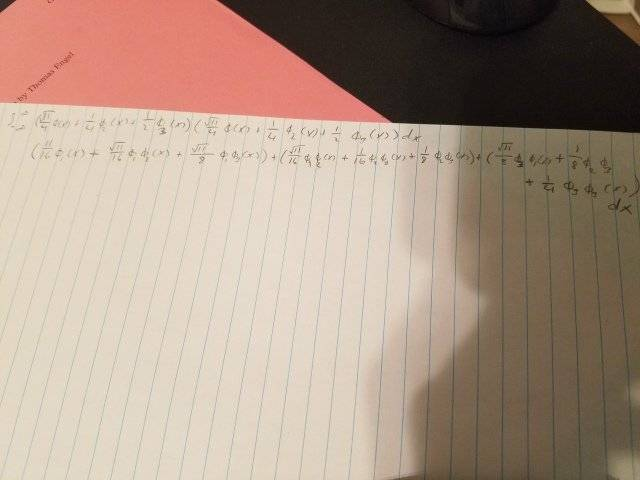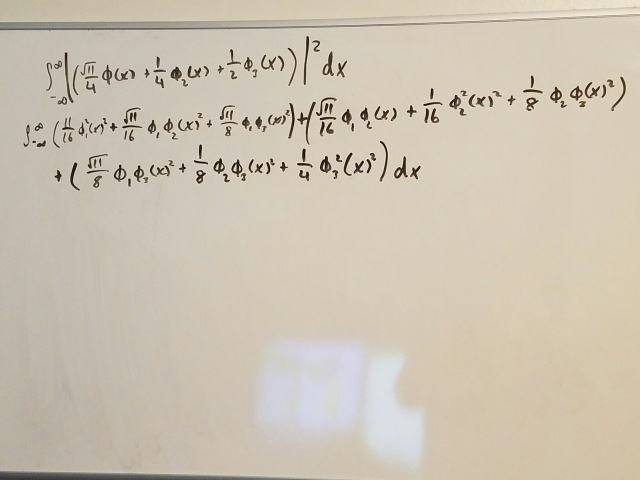# Show that the wave function is normalized

## Homework Statement

Show that the 3 wave functions are normalized

## Homework Equations## The Attempt at a Solution[/B]
The above image is my initial attempt at the first equation.
I was told the way to find out if its normalized is to use:

##\int^{+\infty}_{-\infty} \Psi^2 dx## = 1

but I think I'm misunderstanding part of the math involved. There's an example on Chegg with the problem solved, but in their example the variables that didn't square become zero after integration. And then, the remaining numbers become ... 1. Somehow.

Dr Transport
Gold Member
the eigen functions $\phi_i(x)$ are orthonormal to each other, then you can do the integrals by inspection. The correct expression for the normalization condition is $\int^{\infty}_{-\infty} \left| \Psi(x)\right|^2 dx$

I remembered I had a white board in the living room! Makes this slightly easier to show. This is me redoing the poor photo from before:Is this starting point correct? I feel like I'm going to have a mess when I start integrating.

PeroK
Homework Helper
Gold Member
2020 Award
Is this starting point correct? I feel like I'm going to have a mess when I start integrating.

If you understand the integral as an inner product, there is no need to integrate anything.

Dr Transport
Gold Member
I think you are conceptually having problems with the notation for example it is $\phi_1^*(x)\phi_2(x)$ not $\phi_1^* \phi_2 (x)^2$

I think you are conceptually having problems with the notation for example it is $\phi_1^*(x)\phi_2(x)$ not $\phi_1^* \phi_2 (x)^2$

Thank you for pointing that out. Unfortunately, even though I take notes in class and attempt to read the textbook, I just don't follow some things. The user PeroK mentioned inner products. I haven't heard this term in this class and if I learned it in Calculus its long forgotten. Google showed me this : http://mathworld.wolfram.com/InnerProduct.html , but I don't understand how to apply it this problem. Does this mean that the terms that are the same after the multiplication cancel out?

Dr Transport
Gold Member
You just have to know that $\int^{\infty}_{-\infty} \phi_i^*(x)\phi_j(x) dx = \delta_{ij}$ is the definition of an inner product in space for ortho-normal functions. You should have seen this in your class. There is a general expression for the normalization coefficients, but I'll wait to get it to you, if you don't know what we have said so far, then it won't make sense to you.

vela
Staff Emeritus
Homework Helper
When you say the wave functions are orthonormal, you have to have some concept of orthogonality. What does it mean when you say ##\phi_1## and ##\phi_2##, for example, are orthogonal to each other?

Recall plain old vectors in ##\mathbb{R}^3##. Two vectors ##\vec{x}## and ##\vec{y}## are orthogonal to each other when ##\vec{x}\cdot\vec{y} = 0##. The dot product here is what's called an inner product. The common definition of the dot product results in a concept of orthogonality that coincides with our intuitive sense of two vectors being perpendicular, but there are other ways to define an inner product. Each one defines what it means for two vectors to be orthogonal. If you multiply two vectors together using the appropriate inner product, and the product is 0, we say the vectors are orthogonal.

Depending on the type of vector space you're working with, you have different definitions of an inner product. In the context of quantum mechanics, the inner product of two functions ##f## and ##g## is defined as
$$\langle f, g \rangle = \int_{-\infty}^{\infty} f^*(x)g(x)\,dx.$$ When you say ##\phi_1## and ##\phi_2## are orthogonal, you're saying
$$\int_{-\infty}^{\infty} \phi_1^*(x)\phi_2(x)\,dx = 0.$$

•PeroK
I was able to meet with my professor this morning and he cleared up the issues I was having. Thank you for your help!